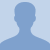# Konst Math: CirclesA circle is a simple shape of Euclidean geometry that is the set of all points in a plane that are at a given distance from a given point, the centre. The distance between any of... A circle is a simple shape of Euclidean geometry that is the set of all points in a plane that are at a given distance from a given point, the centre. The distance between any of the points and the centre is called the radius. It can also be defined as the locus of a point equidistant from a fixed point. A circle is a simple closed curve which divides the plane into two regions: an interior and an exterior. In everyday use, the term "circle" may be used interchangeably to refer to either the boundary of the figure, or to the whole figure including its interior; in strict technical usage, the circle is the former and the latter is called a disk.
More... Collapse
108 Views

## Comments (0)

Please log in to post comments.